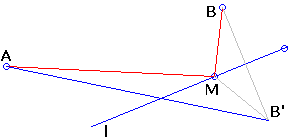# Heron's Problem: What is it? A Mathematical Droodle

Explanation### Heron's Problem

This one is a basic optimization problem. It's quite famous, being discussed in Heron's Catoptrica (On Mirrors from the Greek word Katoptron Catoptron = Mirror) that, in all likelihood, saw the light of day some 2000 years ago.

Two points A and B are given on the same side of a line l. Find a point M on l such that the sum of distances from A and B to M is minimal.Think of l as a mirror. Heron posed that the shortest distance between A and B via l is exactly the path traversed by a ray of light emitted from A and observed at B. From here he deduced that when light is reflected in a mirror the angle of incidence fi is equal to the angle of reflection fr, where the angles at hand are formed by AM and MB with the perpendicular to l at M.

Why so?Let B' be the symmetric image of B in l, such that BB' is perpendicular to l and is divided by the latter in halves. MB = MB', for any point M on l. Therefore, AM + MB = AM + MB'. By the triangle inequality ( ΔAMB'),

AM + MB' ≥ AB'.

with the equality reached only when M lies on AB', in which case clearly the two angles coincide.

Heron's problem has applications to curved surfaces, ellipse for one.

Note: the problem has been known in Japan. It appeared on a now lost sangaku tablet from 1830 written in the Yamagata prefecture [Fukagawa and Pedoe, problem 4.1.1]. The Japanese variant underscored the computational aspects of the solution:

P is a variable point in the given segment AB, and C is a fixed point on the perpendicular AC to the line AB, and D is fixed, on the same side of AB as C, and lies on the perpendicular BD to AB. AC = c, BD = d, and AB = k. Find AP = p such that CP + PD is a minimum when P moves on AB.

It is not difficult to surmise the solution from the diagramWhen P satisfies the minimality requirement, triangles APC and BPD' are similar from which

AP / AC = BP /BD = (AP + BP) / (AC + BD),

or,

p / c = k / (c + d),

so that

p = kc / (c + d).

### References

1. H. Fukagawa, D. Pedoe, Japanese Temple Geometry Problems, The Charles Babbage Research Center, Winnipeg, 1989

Write to:

Charles Babbage Research Center
P.O. Box 272, St. Norbert Postal Station
Winnipeg, MB

2. P. J. Nahin, When Least Is Best, Princeton University Press, 2007 (Fifth printing).
3. V. M. Tikhomirov, Stories about Maximua and Minima, AMS & MAA, 1990
4. D. Wells, The Penguin Book of Curious and Interesting Geometry, Penguin, 1991
5. I. M. Yaglom, Geometric Transformations I, MAA, 1962## Sangaku•